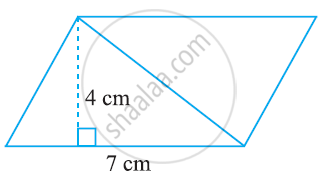# Find the Area of the Following Parallelograms -1 - Mathematics

#### Question

Find the area of the following parallelograms#### Solution

Area of parallelogram = Base × Height

Height= 4 cm

Base = 7 cm

Area of parallelogram = 7 × 4 = 28 cm2

Is there an error in this question or solution?

#### APPEARS IN

NCERT Solution for Mathematics for Class 7 (2018 (Latest))
Chapter 11: Perimeter and Area
11.2 | Q: 1.1 | Page no. 216

#### Video TutorialsVIEW ALL 

Find the Area of the Following Parallelograms -1 Concept: Area of a Parallelogram.